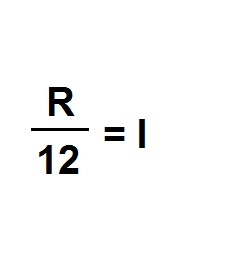Formula - Compounding Interest | Propertylogy
Don't Miss

# Formula – Compounding Interest

By on August 19, 2018

Compounding interest basically means that interest is charged on interest on top of the principal.

One of the biggest secrets of savings and investing is compounding.

Before computing compounding interest, it is important to determine the period or compounding.

For example a loan with monthly compound would have 12 periods a year. While a loan with daily compound will have 365 periods a year.R/12 = I

Where R = Monthly interest

12 = 12 months in a year

I = Monthly interest rate

If the annual interest is 6%, the monthly rate would be 0.5%.

Thus, 0.05/12 = 0.0042

To calculate the total interest of the year, we need to add 1 to 0.5% and multiply it to itself 12 times.

(1+0.0042)12 = 1.0516

For a loan of \$100,000, the result would be a principal growth to \$105,160 at the end of the year.

As you can observe, compounding interest rates will eventually be higher than simple interest.

So make a mental note of that the next time you review your loans.

You May Also Like...##### How to burn more calories in the office

Send this to a friend